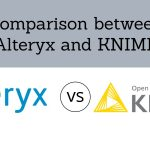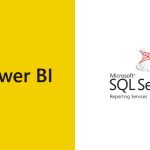[email protected] +1 203-349-9909 / +91-8080042523

ABS Function

### How to use AVERAGEIFS FUNCTION in Excel

The AVERAGEIFS Function of Excel computes the average of the figures in a range that meet one or more delivered conditions. The criteria of the AVERAGEIFS Function also supports the logical operators and wildcard operators in Excel. What Is The Averageifs Function In Excel? AVERAGEIFS Function averages cells in a range that is equivalent to...

### How to use AVERAGEIF FUNCTION

The AVERAGEIF Function of Excel calculates the average of the numbers in a range that meet the supplied conditions. The measures for AVERAGEIF Function supports logical operators and wildcards for partial matching. What is an AVERAGEIF Function in Excel? What is the formula for AVERAGEIF Function? What is the example of AVERAGEIF Function? How is...

### How to use ABS Function in Excel

An Excel Function is a predefined formula that executes the actions like calculating values in a precise order. The common functions of Excel are AGGREGATE, AVERAGE, COUNT, INDEX, ROUND, SUM etc. In this blog, we are going to see all the Excel Functions one by one in depth. Also, you will learn the different parts of a function and how to...